Queries for Integer partitions: search statistic / browse statistics / browse maps from / browse maps to

# 1. Definition & Example

• An integer partition $\lambda$ of $n \in \mathbb{N}_+$ is a sequence $\lambda = (\lambda_1,\ldots,\lambda_k)$ such that $\lambda_i \in \mathbb{N}_{+}$, $\lambda_1 \geq \lambda_2 \geq \dots \geq \lambda_k$ and $\sum_{1 \leq i \leq k} \lambda_i = n$.

• We write $\lambda \vdash n$ if $\lambda$ is a partition of $n$, and denote the set of integer partitions of $n$ by $\mathbf{P}_n$.

 the 7 Integer partitions of size 5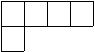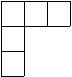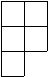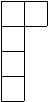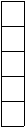[4,1] [3,2] [3,1,1] [2,2,1] [2,1,1,1] [1,1,1,1,1]
• Integer partitions are graphically represented by their Ferrers diagram (or Young diagram) as a collection of boxes.

• The number of integer partitions $p(n) = |\mathbf{P}_n|$ is A000041, and the number of integer partitions into distinct parts is A000009. Its generating function is

• $$\displaystyle \sum_{n=0}^\infty p(n) x^n = \prod_{k=1}^\infty \frac{1}{1-x^k}.$$

This is also known as the Euler's generating function which allows $p(n)$ to be calculated for any $n$, see [Wil00].

# 2. Properties

• Let $p_n(r)$ be the number of partitions of $n$ in which the largest part is $r$. Let $q_n(r)$ be the number of partitions of $n-r$ which no part is greater than r. Then

$$p_n(r)=q_n(r)$$

• Let $p_n^s$ denote the number of self conjugate partitions of n and let $p_n^t$ be the number of partitions of n into distinct odd parts. Then

$$p_n^s=p_n^t$$

• Let $p_n^o$ be the number of partitions of n into odd parts, and let $p_n^d$ be the number of partitions of n into distinct parts. Then

$$p_n^o=p_n^d,$$

• see [Bru10].

• The elements of $\mathbf{P}_n$ index the number of irreducible representations of the symmetric group $\mathbf{S}_n$ over a field of characteristic $0$.

• The number of non-isomorphic abelian groups of order $n=\sum_ip_i^{j_i}$ where $p_i$ are distinct primes and $j_i$ are positive integers is given by $\prod_ip(j_i)$ A000688

• Congruence partitions are partitions that are similar under modulation. For example: $$p(5k+4)= 0 (mod\text{ }5)$$ $$p(7k+5)= 0 (mod\text{ }7)$$ $$p(11k+6)=0 (mod\text{ }11)$$ are just a couple examples proven by Srinivasa Ramanujan.

• The approximation formula for p(n): $$\displaystyle p(n) \sim \frac {1} {4n\sqrt3} \exp\left({\pi \sqrt {\frac{2n}{3}}}\right) \mbox { as } n\rightarrow \infty$$

• For many combinatorial statistics and bijections see [Pak02]

• [Bru10]   R. Brualdi, Partition numbers, Combinatorics Fifth Edition (2010).

[Pak02]   I. Pak, Partition bijections, a survey, Ramanujan J. 12(1) (2006) p. 5-75.

[Wil00]   S. Wilf, Basic generating functions, Lectures on Integer Partitions (2000).

# 5. Technical information for database usage

• An integer partition is uniquely represented as a list of its parts.
• Integer partitions are graded by their sum.
• The database contains all integer partitions of size at most 17.